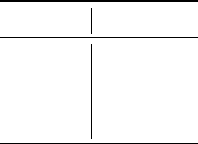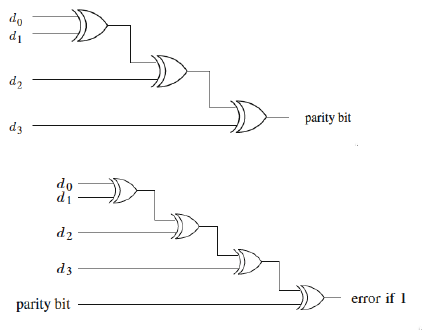1
Solution 1.1. R = R
1
R
2
R
3
et R
dup
= [1(1R
1
)
2
][1(1R
2
)
2
][1(1R
3
)
2
]
2
Solution 2.1. R = R
1
R
2
R
3
et R
dup
= [1 (1 R
1
R
2
R
3
)
2
]
3
Solution 3.1. The obtained expressions show that ﬁne grain redundancy leads
to higher reliability.
4
Solution 4.1.
x
1
x
2
x
3
ξ(x)
0 0 0 0
0 0 1 0
0 1 0 0
0 1 1 1
1 0 0 0
1 0 1 1
1 1 0 1
1 1 1 1
with x = {x
1
, x
2
, x
3
}
The path vectors are (011), (101), (110) and (111).
The minimal path vectors are (011) (101) and (011).
Then, the state function is:
ξ(x) = 1 (1 x
1
x
2
)(1 x
2
x
3
)(1 x
1
x
3
)
= 1 (1 x
1
x
2
)(1 x
1
x
3
x
2
x
3
+ x
1
x
2
x
3
)
= 1 (1 x
1
x
3
x
2
x
3
+ x
1
x
2
x
3
x
1
x
2
+ x
1
x
2
x
3
+ x
1
x
2
x
3
x
1
x
2
x
3
)
= x
1
x
3
+ x
2
x
3
+ x
1
x
2
2x
1
x
2
x
3
The general expression of the reliability is R = R
1
R
3
+ R
2
R
3
+ R
1
R
2
+
2R
1
R
2
R
3
If all modules have the same reliabilty R
M
, it becomes R = 3R
2
M
2R
3
M
.
Another way to ﬁnd this expression is noting that the system functions for
the case when all modules function (this leads to R
3
) and for the three cases
when two modules function and one module fails (this leads to 3(1 R
M
)R
2
M
):
R = R
3
M
+ 3(1 R
M
)R
2
M
= R
3
M
+ 3(R
2
M
R
3
M
)
= 3R
2
M
2R
3
M
1Solution 4.2. R = (3R
2
M
2R
3
M
)R
v
R R
M
(3R
2
M
2R
3
M
)R
v
R
M
(3R
M
2R
2
M
)R
v
1
R
v
1
3R
M
2R
2
M
5
Solution 5.1. Yes. Because both functions are self-dual.
Solution 5.2. f
sd
= x
n+1
f(x
1
, · · · , x
n
) + x
n+1
f(x
1
, · · · , x
n
)
6
Solution 6.1. There are three cases.
The sum bit s
i
ˆ
S = S 2
i
if faulty ˆs
i
= 0 or
ˆ
S = S + 2
i
if faulty ˆs
i
= 1
The carry-out bit c
i+1
ˆ
S = S 2
i+1
if faulty
ˆc
i+1
= 0 or
ˆ
S = S + 2
i
if faulty ˆc
i+1
= 1
Both sum bit s
i
and c
i+1
are aﬀected: there are four possibilities:
ˆc
i+1
ˆs
i
ˆ
S = S
0 0 3 × 2
i
0 1 2
i
1 0 2
i
1 1 3 × 2
i
So the result will diﬀer of one of the values 0, ±2
i
, ±3 × 2
i
, ±2
i+1
Solution 6.2. There are three cases.
The sum bit is aﬀected: the addition
ˆ
S = S 2
i1
if faulty ˆs
i
= 0 or
ˆ
S = S + 2
i1
if faulty ˆs
i
= 1
The carry-out bit is aﬀected: the addition
ˆ
S = S 2
i
if faulty ˆc
i+1
= 0 or
ˆ
S = S + 2
i
if faulty ˆc
i1
= 1
Both sum bit s
i2
and c
i1
are aﬀected: there are four possibilities:
ˆc
i+1
ˆs
i
ˆ
S = S
0 0 3 × 2
i1
0 1 2
i1
1 0 2
i1
1 1 3 × 2
i1
2So the result will diﬀer of one of the values 0, ±2
i1
, ±3 × 2
i1
, ±2
i
Solution 6.3. There are three cases.
The sum bit is aﬀected: the addition
ˆ
S = S 2
i2
if faulty ˆs
i
= 0 or
ˆ
S = S + 2
i2
if faulty ˆs
i
= 1
The carry-out bit is aﬀected: the addition
ˆ
S = S 2
i1
if faulty ˆc
i+1
= 0
or
ˆ
S = S + 2
i1
if faulty ˆc
i1
= 1
Both sum bit s
i2
and c
i1
are aﬀected: there are four possibilities:
ˆc
i+1
ˆs
i
ˆ
S = S
0 0 3 × 2
i2
0 1 2
i2
1 0 2
i2
1 1 3 × 2
i2
So the result will diﬀer of one of the values 0, ±2
i2
, ±3 × 2
i2
, ±2
i1
We can conclude that to detect the errors, we must consider two bit shift
because one bit shift is not enough. Indeed, except when both results are exact,
no-shift and two-bit shift calculations will lead to diﬀerent results. Nevertheless,
the results of no-shift and one-bit shift calculations will be the same in two cases
(diﬀerence 0 and diﬀerence ±2
i
).
7
Solution 7.1. A parity code can detect single-bit errors and multiple-bit errors
which aﬀect an odd number of bits.
If an odd number of bits is aﬀected, then the number of 1’s in a codeword
changes from even to odd (for an even parity code) or from odd to even
(for an odd parity code).
A parity code can only detect errors, but it cannot correct them, since the
position of the erroneous bit(s) is not possible to locate.
For example, suppose that we use an even parity code to protect the trans-
mission of 5-bit data. If we receive the word , then we know that
an error has occurred. However, we do not know which codeword has been
sent. If we assume that a single-bit error has occurred during the trans-
mission, then it is equally likely that one of the six codewords ,
, , , , or  has been sent.
Solution 7.2. The decision depends on which type of all-bits error is more
probable.
For even parity - the parity bit for the all zeroes data word will be 0 and an
all-0’s failure will go undetected - it is a valid codeword.
3Selecting the odd parity code will allow the detection of the all-0’s failure.
If all-1’s failure is more likely - the odd parity code must be selected if the
total number of bits (d + 1) is even, and the even parity if d + 1 is odd
Solution 7.3.
Solution 7.4.
8
Solution 8.1. Berger codes can detect any number of one-to-zero bit-ﬂip errors,
as long as no zero-to-one errors occurred in the same code word. Similarly,
Berger codes can detect any number of zero-to-one bit-ﬂip errors, as long as no
one-to-zero bit-ﬂip errors occur in the same code word. Berger codes cannot
correct any error.
9
Note there was an error on matrix G (see bold values)!
G =
1 0 0 0 1 1
0 1 0 1 0 1
0 0 1 1 1 0
Solution 9.1. We note that G = [I
k
A], then we can get H = [A
T
I
nk
]
H =
0 1 1 1 0 0
1 1 0 0 1 0
1 0 1 0 0 1
4